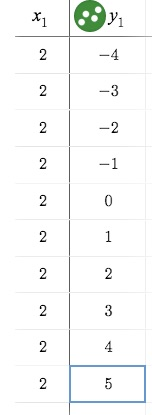# LINR 1 | Lesson 3 | Explore (Vertical Lines) Solutions

2.$$x$$ values are all the same
3. Change in $$\frac{Δy}{Δx}= \frac{1}{0}$$
5. There is no $$y$$-intercept
6. Equation is $$x = 2$$